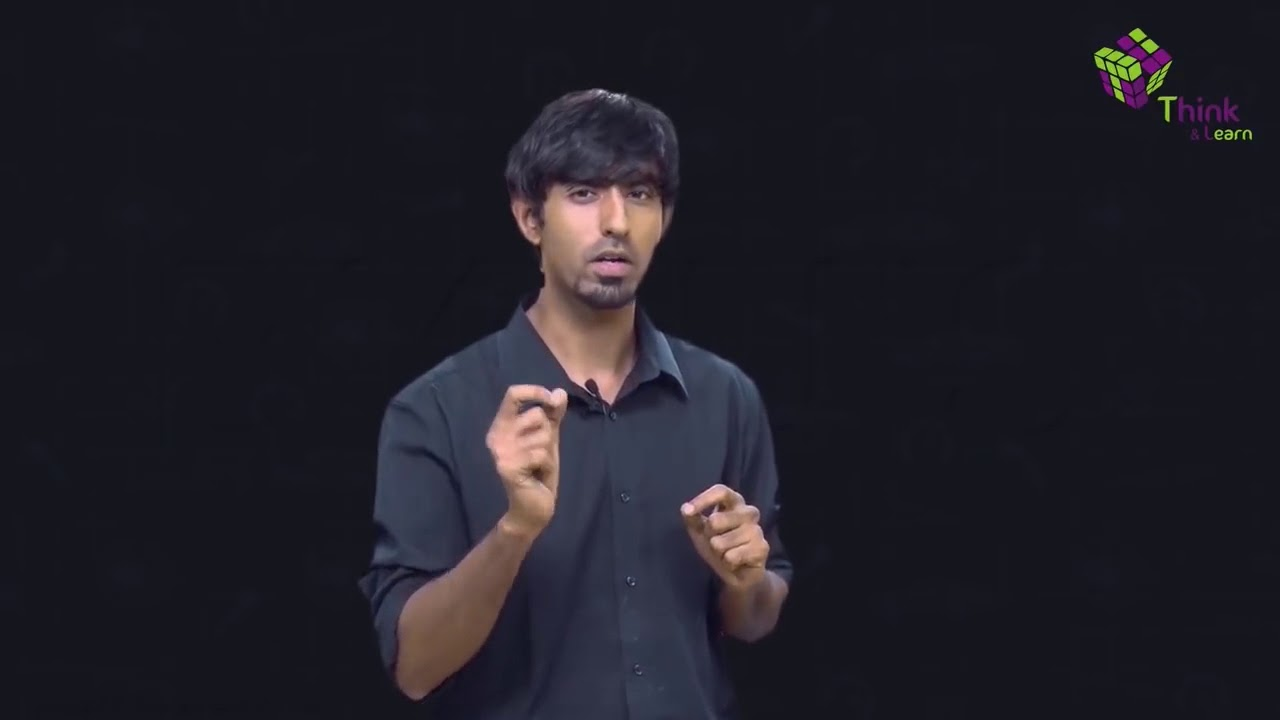# A racing car has a uniform acceleration of 4m/s square. What distance will it cover in 10sec after start?

A racing car has a uniform acceleration of 4m/s square, it will cover 200m in 10sec after start.

### Given

• The initial velocity (u) = 0
• The acceleration (a) = 4m/s
• Time (t) = 10 sec

### Need to find

The final velocity (v) = ?

### Solution

Motion is the phenomenon in which an object changes its position. The first equation of motion is given as:

v = u + at

Where,

• v = final velocity
• u = initial velocity
• a = acceleration
• t = time taken

Substitute the given parameters in the above equation.

v = 0 + 4 × 10

v = 0 + 40

v = 40m/s

The third equation of motion is given as:

=

Where,

• v = final velocity
• u = initial velocity
• a = acceleration
• t = time taken

Substitute the known parameters in the above equation.

(40)2 = 2 x 4 x s

1600 = 8s

s = 1600/8

s = 200 m

∴ Distance it will cover in 10sec after start is 200mArticles to explore(171)(21)